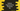# Trigonometric functions in python## Introduction :

Finding out the trigonometric value like sine, cosine or tangent will be hard if we don’t use any package or library. Almost every programming language provides trigonometric functions. For python, these are defined in the math module. math module in Python provides different types of mathematical functions defined by the C standard. In this post, I will show you the list of all trigonometric functions defined in python math module with examples :

## List of trigonometric functions :

Following are the list of all trigonometric functions defined in math :

### 1. math.sin(x) :

It returns the sine of x, where x is in radians.

### 2. math.cos(x) :

It returns the cosine of x, where x is in radians.

### 3. math.tan(x) :

It returns the tangent of x, where x is in radians.

### 4. math.asin(x) :

It returns the arc sine of x, where x is in radians.

### 5. math.acos(x) :

It returns the arc cosine of x, where x is in radians.

### 6. math.atan(x) :

It returns the arc tangent of x, where x is in radians.

### 7. math.atan2(y, x) :

It returns atan(y/x). The return value is in radians and the result is always between -pi and pi.

### 8. math.dist(x,y) :

It returns the Euclidean distance between two points x and y.

### 9. math.hypot(*values) :

It returns the Euclidean norm or the length of the vector from the origin to this point. In two dimensional plan, it is equal to sqrt(aa + bb) for a point (a, b). Similarly, for an n-dimensional plan, it is equal to the sum of squares of all points and the square root of this value.

## Sample program :

``````import math

degree = float(input("Enter the value in degrees : "))
radian = degree * 0.0174533

print("sin({}) : {}".format(degree, math.sin(radian)))
print("cos({}) : {}".format(degree, math.cos(radian)))
print("tan({}) : {}".format(degree, math.tan(radian)))``````

### Output :

``````Enter the value in degrees : 30
sin(30.0) : 0.5000001943375613
cos(30.0) : 0.8660252915835662
tan(30.0) : 0.5773505683919328

Enter the value in degrees : 60
sin(60.0) : 0.8660256281860526
cos(60.0) : 0.499999611324802
tan(60.0) : 1.7320526027838818

Enter the value in degrees : 45
sin(45.0) : 0.7071070192004544
cos(45.0) : 0.7071065431725606
tan(45.0) : 1.0000006732053301``````